# FSK-Modulated RF Subcarrier Fiber-Optic Transmission

## Tool Used: OptSim

This application note demonstrates transmission of an FSK-modulated RF sub-carrier over standard singlemode fiber.

Subcarrier multiplexing helps carry multiple RF data streams over a single optical carrier, thereby saving on the number of lasers. The RF data can be amplitude modulated (ASK), or frequency modulated (FSK) or phase modulated (PSK). The modulated RF is then used to modulate optical carrier. The detected electrical signal at the receiver is demodulated using the appropriate demodulation technique depending on the type of modulation used at the transmitter. This application note uses frequency modulation of the RF signal.

Figure 1 shows the OptSim schematic layout using direct and external modulation of the laser.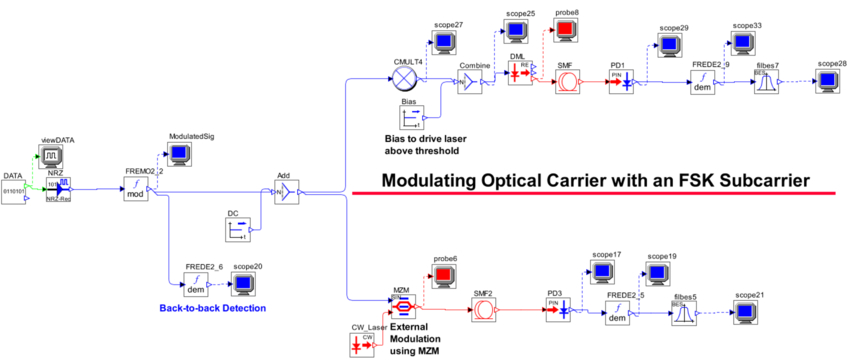The output of the frequency modulator model is: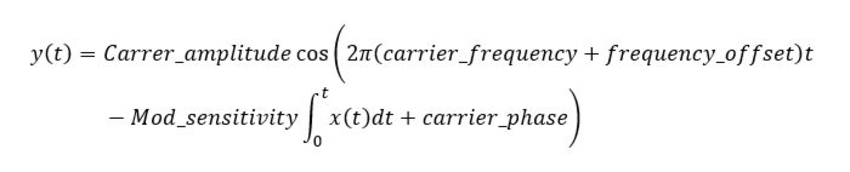The term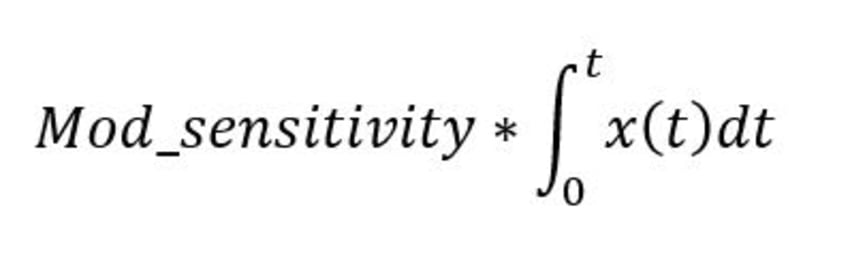gives carrier phase deviation caused by x(t), and the derivative of this phase term gives the deviation in instantaneous frequency (Mod_sensitivity*x(t)).

The RF carrier at 10GHz is amplitude modulated by the digital data signal carrying 1Gbps binary data.

Figure 2 shows FSK modulated RF carrier.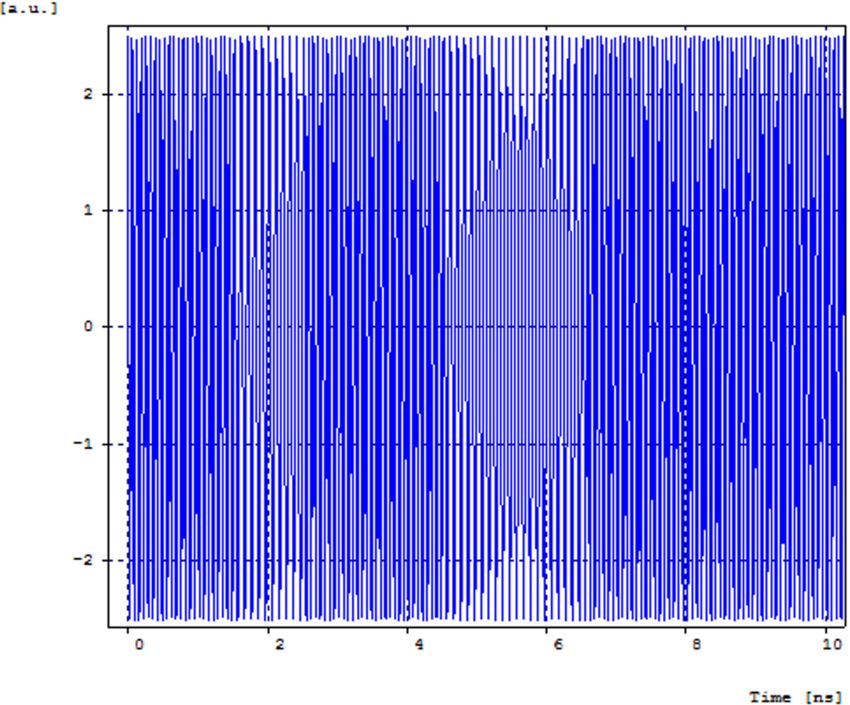Figure 2. FSK modulated RF carrier

The spectrum of the FSK-modulated RF is shown in Figure 3.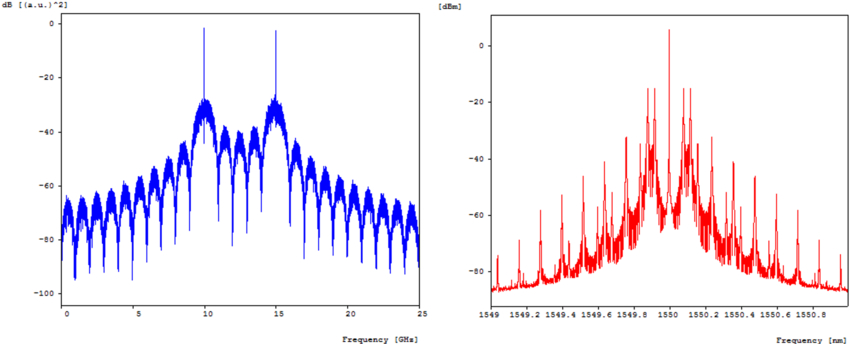Figure 3. FSK-modulated RF spectrum (left) and spectrum of the RF-modulated optical carrier (right)

The FSK-modulated RF subcarrier is used to modulate an optical carrier at 1550 nm. Two configurations are shown: one uses direct modulation of the laser, and the other uses MZM-based external modulation of the laser. The signal travels through a 10 km long single-mode fiber. The detected baseband signal at the receiver is demodulated and low-pass filtered to get demodulated data signal.

Figure 4 shows detected data at the output of the low-pass filter (left) and corresponding eye diagram (right).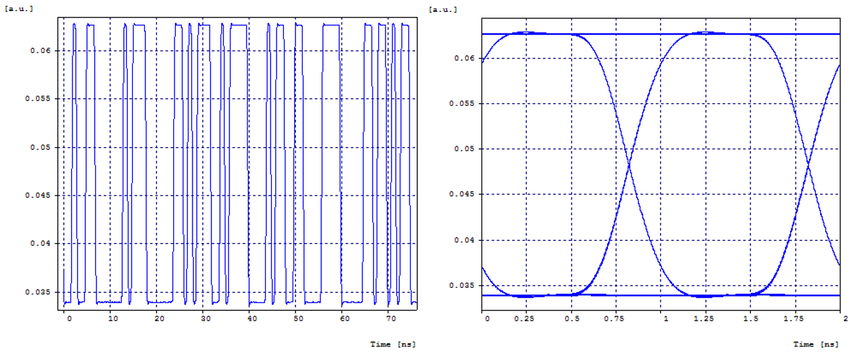Figure 4. Detected data (left) and eye diagram (right)

The eye diagram is wide open due to relatively short transmission distance though FSK-modulated links are not suitable for longer distances due to higher penalties due to fiber dispersion.